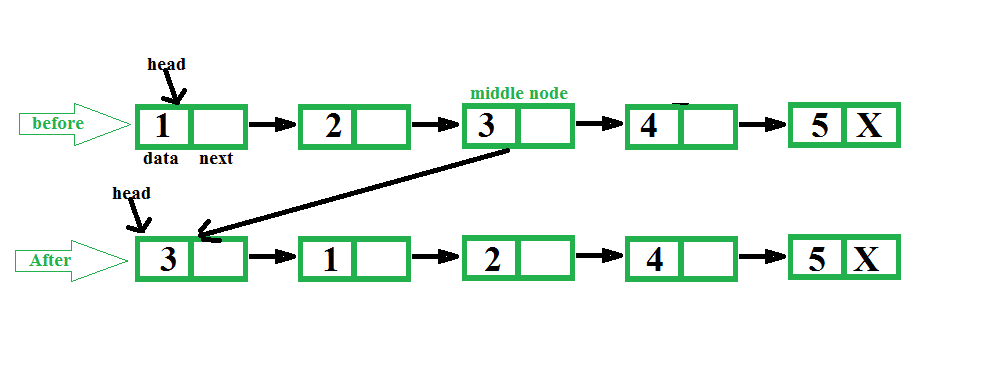Open In App

# C Program For Making Middle Node Head In A Linked List

Given a singly linked list, find middle of the linked list and set middle node of the linked list at beginning of the linked list.
Examples:

```Input: 1 2 3 4 5
Output: 3 1 2 4 5

Input: 1 2 3 4 5 6
Output: 4 1 2 3 5 6 ```The idea is to first find middle of a linked list using two pointers, first one moves one at a time and second one moves two at a time. When second pointer reaches end, first reaches middle. We also keep track of previous of first pointer so that we can remove middle node from its current position and can make it head.

## C

 `// C program to make middle node as``// head of linked list.``#include ``#include ` `// Link list node``struct` `Node``{``    ``int` `data;``    ``struct` `Node* next;``};` `/* Function to get the middle and set at``   ``beginning of the linked list*/``void` `setMiddleHead(``struct` `Node** head)``{``    ``if` `(*head == NULL)``        ``return``;` `    ``// To traverse list nodes one by one``    ``struct` `Node* one_node = (*head);` `    ``// To traverse list nodes by skipping``    ``// one.``    ``struct` `Node* two_node = (*head);` `    ``// To keep track of previous of middle``    ``struct` `Node* prev = NULL;``    ``while` `(two_node != NULL &&``           ``two_node->next != NULL)``    ``{``        ``// For previous node of middle node``        ``prev = one_node;` `        ``// Move one node each time``        ``two_node = two_node->next->next;` `        ``// Move two node each time``        ``one_node = one_node->next;``    ``}` `    ``// Set middle node at head``    ``prev->next = prev->next->next;``    ``one_node->next = (*head);``    ``(*head) = one_node;``}` `// To insert a node at the beginning``// of linked list.``void` `push(``struct` `Node** head_ref,``          ``int` `new_data)``{``    ``// Allocate node``    ``struct` `Node* new_node =``           ``(``struct` `Node*)``malloc``(``sizeof``(``struct` `Node));` `    ``new_node->data = new_data;` `    ``// Link the old list of the new node``    ``new_node->next = (*head_ref);` `    ``// Move the head to point to the``    ``// new node``    ``(*head_ref) = new_node;``}` `// A  function to print a given linked list``void` `printList(``struct` `Node* ptr)``{``    ``while` `(ptr != NULL)``   ``{``        ``printf``(``"%d "``, ptr->data);``        ``ptr = ptr->next;``    ``}``    ``printf``(``""``);``}` `// Driver code``int` `main()``{``    ``// Create a list of 5 nodes``    ``struct` `Node* head = NULL;``    ``int` `i;``    ``for` `(i = 5; i > 0; i--)``        ``push(&head, i);` `    ``printf``(``" list before: "``);``    ``printList(head);` `    ``setMiddleHead(&head);` `    ``printf``(``" list After:  "``);``    ``printList(head);` `    ``return` `0;``}`

Output:

```list before: 1 2 3 4 5
list After : 3 1 2 4 5 ```

Time Complexity: O(n) where n is the total number of nodes in the linked list.

Auxiliary Space: O(1) since using constant space